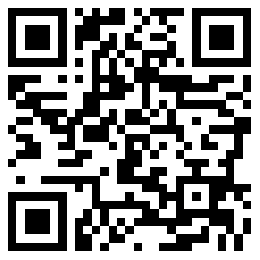## 黄金分析## 脚本下载## 期货知识# EA编程教程第五讲 操作符

admin 外汇智能交易编程 2007-07-27 04:12:01 4334 0

1 复合语句。

if(x==0)

{

Print("invalid position x=",x);

return;

}

2 表达式

i = 4;

i = a = 7; //错误，一个表达式中不能同时出现两个以及两个以上的等号（=）。

3 break 语句。

break语句使程序跳出最近的一个，while，for，switch语句。如果在一个循环语句里面已经完成了需要的工作，则可以使用break语句跳出循环。例如：

for(a=0;a

if((array[a]==0)

break;

4 continue

continue用于结束执行当前循环语句体，直接进入下一个循环，可用在for和while循环体语句中。例如:

int a = 0;

for(int j = 0; j < 10; j++)

{

if (array[j] <= 0)

{

continue; //遇到continue，则程序进入下一个循环，不会再执行 a += array[j] 语句,注意此时不是跳出循环体。}

a += array[j];

}

5 return

return语句结束当前函数的执行，并返回一个值给调用这个函数的程序，也可以不返回值。例如：

int CalcSum(int x, int y)

{

return(x+y); //函数执行到这里就中断了，返回值为x+y;后面的语句都不会执行了。

int i = x * y; //改语句执行不到了。

}

6 条件语句 if-else

if (a == 0)

{

Print("a");}

else  if (a < 0)

{

Print("小于0");}

else

{

Print("大于0");}

7 switch 语句

int x = 1

switch(x)

{

case 1:

Print("CASE 1");//x的值为1的时候

break;

case 2:

case 3:

Print("CASE 2 or 3"); //x的值为2或3

break;

default:

Print("NOT 1,2,3"); //x的值不是1,2,3

break;

}

switch里面如果程序没有碰到break语句，或继续执行知道碰到break;或者程序块结束。

8 循环语句 while，for

int k = 1;

int n = 10;

while(k

{

Print(k);

k++;

}//将从1打印到10。

for(int n = 0; n < 10; n++)

Print(n); //将从0打印到9

#### 发表评论

☹还没有评论，来说两句吧...

https://wh1828.com/

### | 鄂ICP备100890000